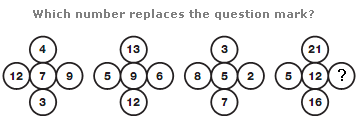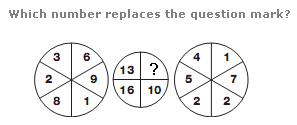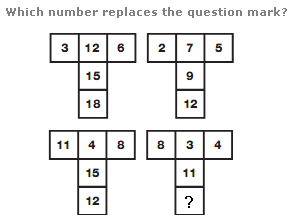# Puzzles - Number puzzles

### Exercise :: Number puzzlesAnswer : 6 Explanation : In each group of circles, the centre number equals the average of the four surrounding numbers.Answer : 11 Explanation : Split the left and right hand circles in half, vertically. The sum of the numbers in the left hand half of the left circle appears in the top left hand segment of the middle circle, and the sum of the numbers in the right hand half of the left hand circle appears in the bottom left hand segment of the middle circle. Repeat this pattern for the right hand circle.Answer : 7 Explanation : In each diagram, the sum of the upper left and upper middle numbers is written in the centre box, and the sum of the upper right and upper middle numbers is written in the lower central box.